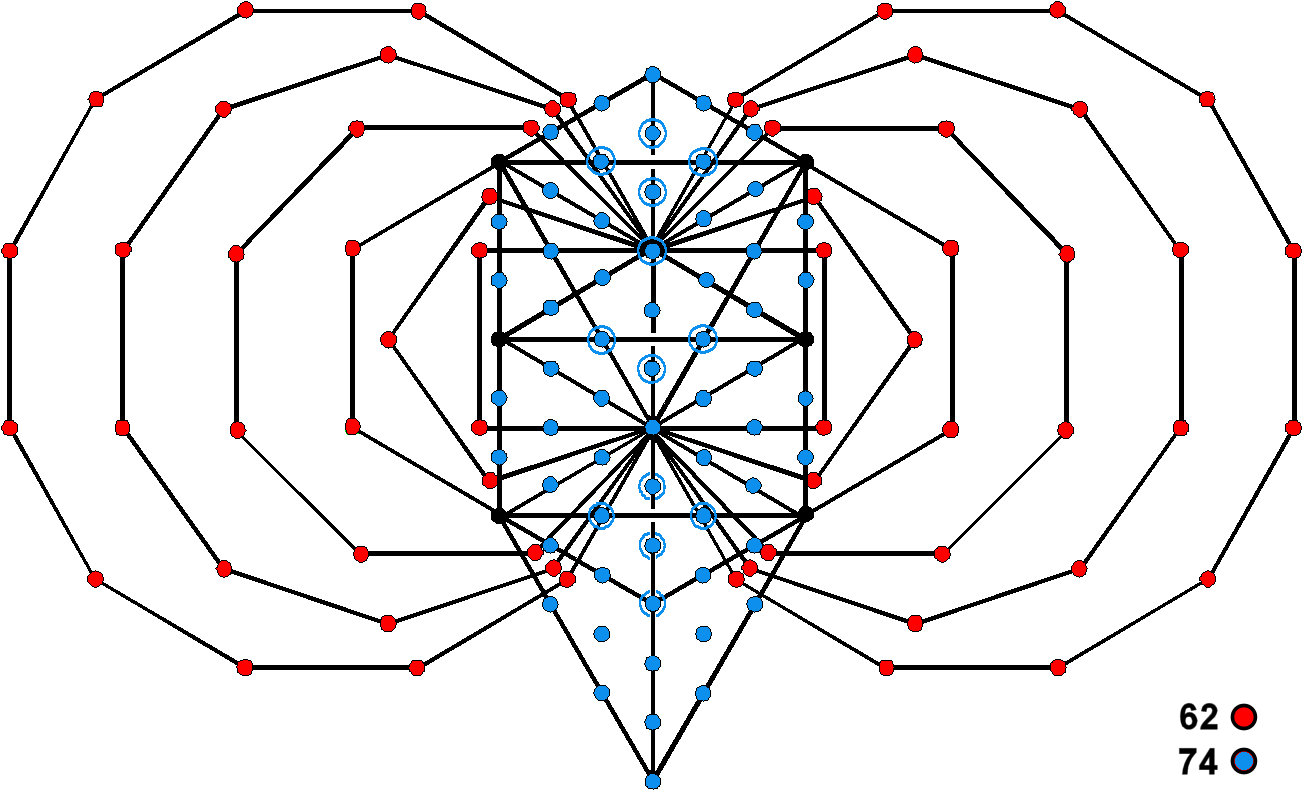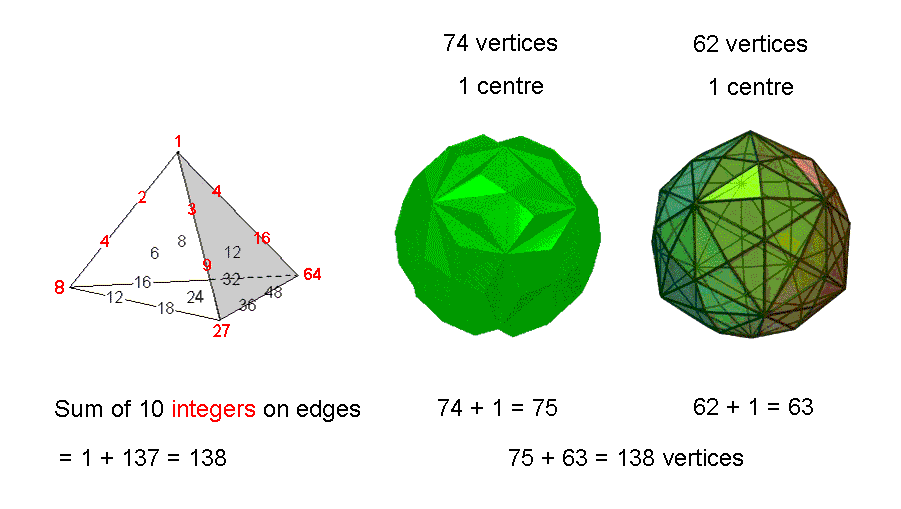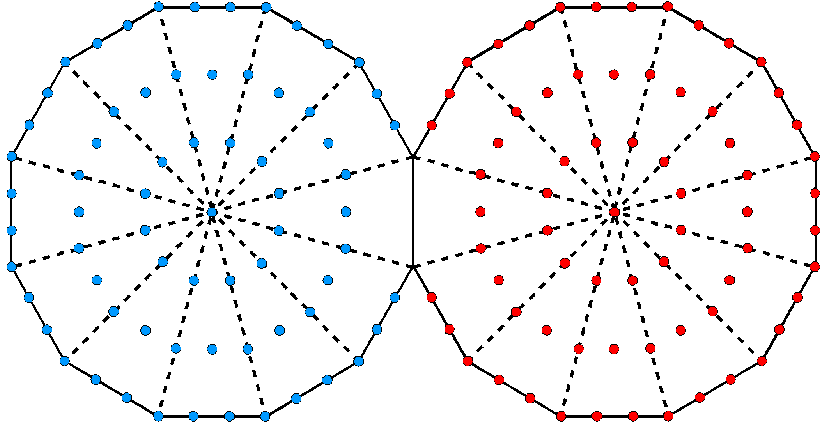<< Previous    1...   7  8    10  11  ...14    Next >>

Equivalence of the Polyhedral Tree of Life & the Tetrahedral Lambda

When the 1-tree (lowest Tree of Life) constructed from tetractyses is superposed on its inner form — the 14 enfolded, regular polygons — the outer corners of the two triangles coincide with Chesed and Geburah and the top and bottom corners of the two hexagons coincide with Chokmah, Binah, Netzach & Hod. Hence, six of the 70 corners of the 14 polygons are shared and 64 corners are unshared. 62 of these corners are outside the root edge. 74 of the 80 yods in the 1-tree are not corners of the polygons, so that (74+62=136)Figure 15. The 62 unshared corners & 74 unshared yods.

yods or corners outside the root edge are unshared (Fig. 15). Their counterpart in the Polyhedral Tree of Life (Fig. 16) are the 74 vertices of the 144 Polyhedron and the 62 vertices of the disdyakis triacontahedron. Their two centres correspond to the endpoints of the root edge. These (74+62+2=138) points are the corners of 216 triangles inside the former polyhedron generated by its 216 edges and of 180 triangles inside the latter generated by its 180 edges, the centre of each polyhedron being the corner shared by each set of triangles. The counterpart in the Tetrahedral Lambda of these 138 points is the fact that 138 is the sum of the 10 red integers (all powers of 2, 3 & 4) that lie on the three edges meeting at the apex to which the number 1 is assigned.Figure 16. Equivalence of the Tetrahedral Lambda and the Polyhedral Tree of Life.

The number 138 is a parameter characterizing holistic systems. It appears in the pair of dodecagons — the last polygons of the inner Tree of Life — as the 138 yods outside their shared root edge (Fig. 17). Notice that 64 yods line their 22 unshared sides. Located at a vertex, this is the largest number in the Tetrahedral Lambda. It is the number value of Nogah, the Mundane Chakra of Netzach (see Table 1).Figure 17. 138 yods make up the two joined dodecagons of the inner Tree of Life outside their shared side.

The number 138 also manifests in the four Platonic solids whose shapes the ancient Greeks thought were those of the particles of the Elements Earth, Water, Air & Fire. When their 38 faces are constructed from tetractyses, 137 yods are needed on average (see here). Therefore, 138 yods on average occupy their faces and centres. The central yod is the counterpart of the integer 1, the Pythagorean Monad, at the apex of the Tetrahedral Lambda. In each case, it signifies the source of the archetypal object.

 << Previous    1...   7  8    10  11  ...14    Next >>

Home